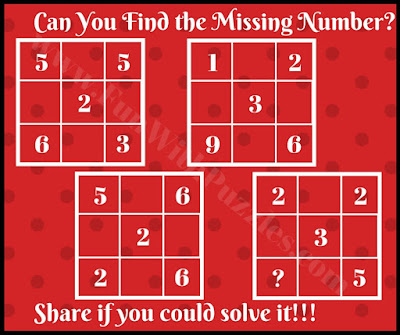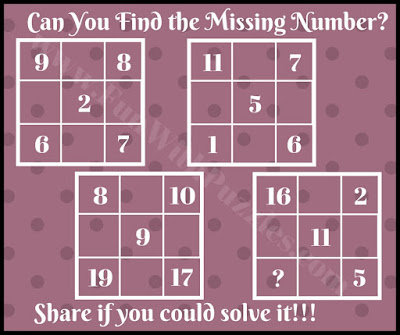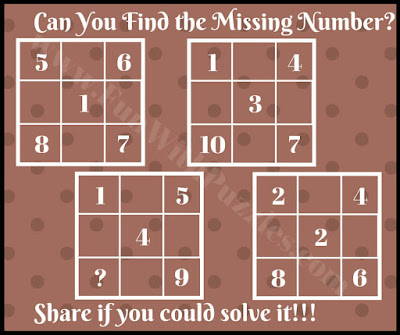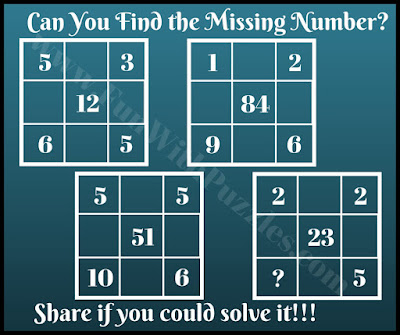Can you find the missing number? Are you ready to find which number will replace the question mark? Here are brain teasers and Maths Picture Puzzles in which you have to find the missing number in each of the maths picture puzzles. You need to find the hidden relationship between the given numbers and then apply it number set in which there is the missing number to find out the number which will replace the question mark.
In these Brain Teasers and Maths Picture Puzzles, there are four groups of five numbers. These five numbers are related to each other by some mathematical operations. Find this hidden relationship to calculate the value of the missing number in one of the sets of five numbers.
Answers to these Missing Number Math Brain Teasers are given at the end. If you come up with a different answer, do post it along with your explanation. It will be interesting to know the different viewpoints to solve these puzzles.1. Can you solve this Missing Number Puzzle?2. Missing Number Picture Math Riddle for Middle School Students3. Can you solve this Awesome Picture Math Riddle?4. Can you solve this challenging Maths Brain Teaser?5. Can you solve this Tricky Picture Math Brain Teaser?

Mathematical Puzzles are a very interesting puzzle type. Do check out similar Maths and Logic puzzles as mentioned below to challenge your brain

## List of Maths and Logic Puzzles

1) Math Brain Teasers for Kids with Answers and Explanations: These are very interesting Mathematical Equation Puzzles in which your challenge is to solve Maths Equations with pictures as variables.

2) Interesting Brain Teasers to Challenge Your Mind: These are very interesting Maths Logic and Picture Puzzles which will twist your mind.

3) Will you crack the code? : In these puzzles, your challenge is to find the 3-digit code to open the lock. These are Logical Puzzles.

4) 5 Challenging Brain Teasers For Adults With Answers and Explanation: These are challenging puzzles in which your challenge is to break the Mathematical pattern to find the missing number.

## Answers to Missing Number Brain Teasers

Answers to these Missing Number Brain Teasers and Math Riddles are given below. Do write in the comments section in case you have any doubts about these brain teasers.

A1. 9

A2. 13

A3. 13

A4. 4

A5. 3
The tens digit in the middle number is the tens digit of the multiplication of the top left and bottom left numbers. Similarly, the units digit is the units digit of the multiplicative result of the top right and bottom right numbers.